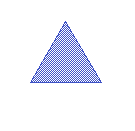Parametric Shape Library

The parametric shape library is a simple library of shapes without any electrical functions. For some shapes it is simpler to create it by entering parameters than to manually draw its layout. This kind of design is called parametric layout and a common selection of designs is shipped with the LayoutEditor in this Parametric Shape Library. Please see below for a list of available shapes.

Each shape consists of a symbol used with the schematic, a parameter definition and a LayoutEditor macro, which will create a layout from the entered parameters. Symbol and parameter definition is stored in the LayoutEditorLibrary format and included with every package of the LayoutEditor. No installation is required to use it. Just choose the shape library from the Components window in the SchematicEditor and place the parametric shape you need.

Alignment Mark

Parameter:

• size
• thickness
• space
• boxsize
• layer_1
• layer_2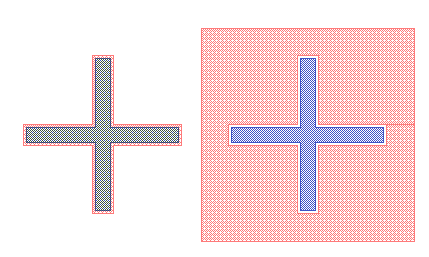Arc Taper

Parameter:

• width1
• width2
• length
• shape
• layer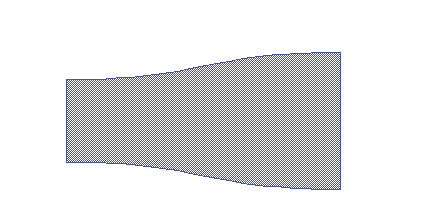Barcode

Parameter:

• width
• height
• text
• code
• layer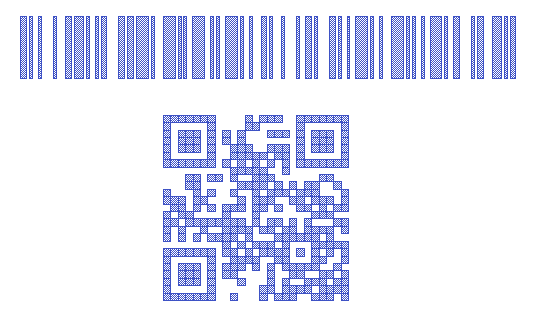Checker Board

Parameter:

• size
• nx
• ny
• layer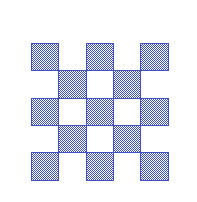Circle

Parameter:

• layer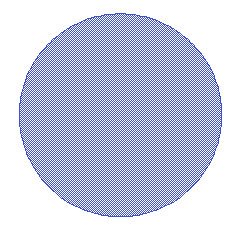Circular Lens

Parameter:

• width
• height
• points
• layer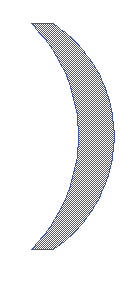Cog Wheel

Parameter:

• layer
• number of teeth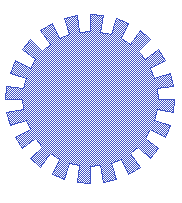Coil

Parameter:

• width
• space
• turns
• layer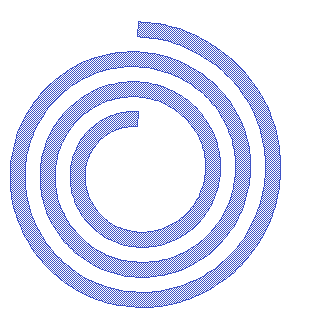Comb

Parameter:

• width
• length
• space
• base
• turns
• layer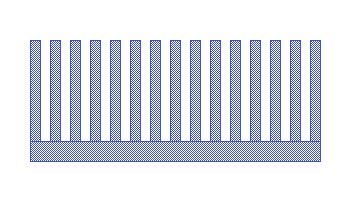Comb Drive

Parameter:

• width
• length
• space
• base
• offset
• turn
• layer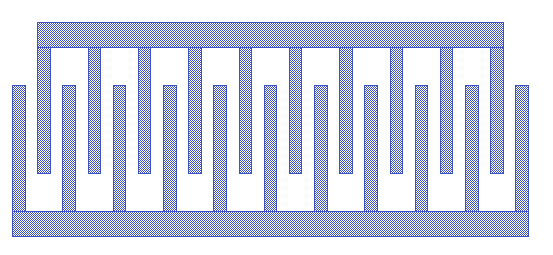Donut

Parameter:

• layer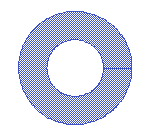Dot Array

Parameter:

• size
• space
• nx
• ny
• layer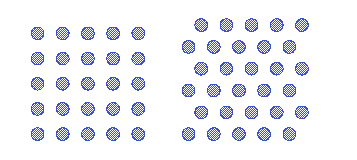Dot Array Invers

Parameter:

• size
• space
• nx
• ny
• layer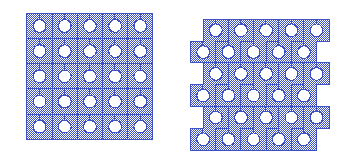Ellipse

Parameter:

• layer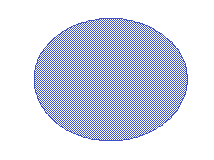Eliptic Donut

Parameter:

• thickness
• layer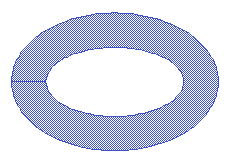Fresnel Zone Plate Lens (zone plate)

Parameter:

• wavelength
• focus
• startWithShape
• layer
• mode
• exact: arcs are cut to achieve exact given radius,
• limited: arcs not fitting in the given radius are not created,
• extended: arcs are created with the original size even if not fitting the given radius.
• startradius, smaller arcs are not created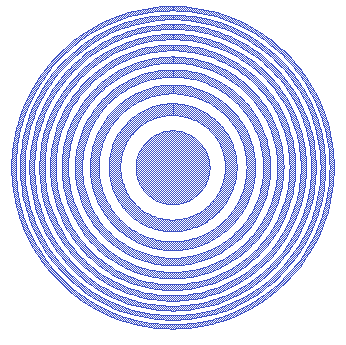Label Array

Parameter:

• label
• textHeight
• spaceX
• spaceY
• nx
• ny
• rowFormat
• colFormat
• layer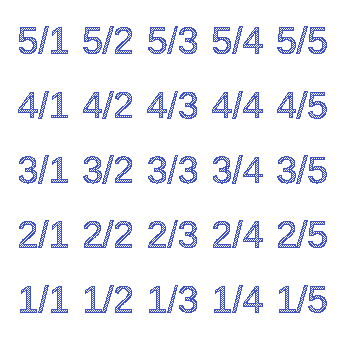Linear Taper

Parameter:

• width1
• width2
• length
• layer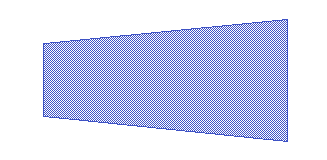Meander

Parameter:

• width
• space
• length
• turns
• layer
•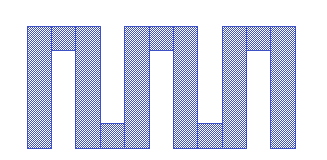Nonius

Parameter:

• size
• nonius
• space
• steps
• layer_1
• layer_2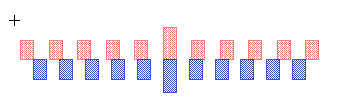Parabolic Lens

Parameter:

• width
• height
• parabol
• points
• layer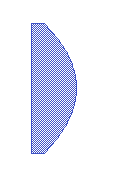Perforated Plate

Parameter:

• width
• length
• holewidth
• holespace
• layer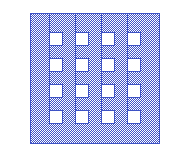Rectangle

Parameter:

• width
• length
• layer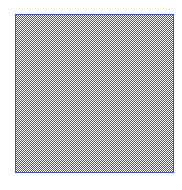Regular Polygon

Parameter:

• layer
• vertexes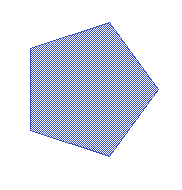S-Blend

Parameter:

• width
• length
• step
• shape
• layer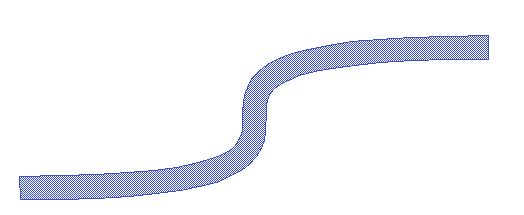Sector

Parameter:

• angle
• layer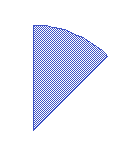Siemens Star

Parameter:

• arcwidth
• arcspace
• layer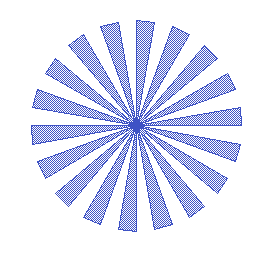Square Array

Parameter:

• size
• space
• nx
• ny
• layer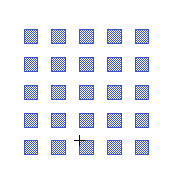Star

Parameter:

• vertexes